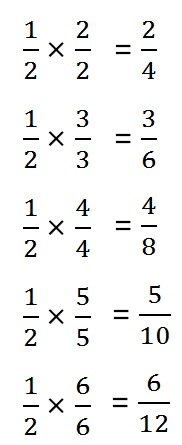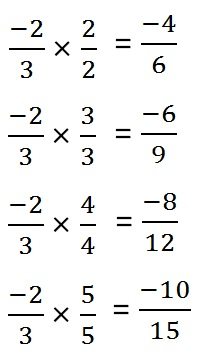Equivalent Rational Numbers

Chapter 8 Class 7 Rational Numbers
Concept wise

For rational number 1/2,

equivalent rational numbers areSo,

equivalent rational numbers are 1/2, 2/4, 3/6, 4/8, 5/10, 6/12

Similarly,

For rational numbers are (-2)/3,

equivalent rational numbers areSo, equivalent numbers are (-2)/3, (-4)/6, (-6)/9, (-8)/12, (-10)/15

Let’s do some questions.

Learn in your speed, with individual attention - Teachoo Maths 1-on-1 Class